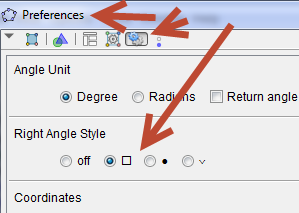# Right angle representation

ilic.ferretti shared this question 6 years ago

I need to underline that two segments are orthogonal, so I would like to show that the angle they form measures 90° degree. I created the angle and it showed up fine as a small square (see first attached image).

At a certain point, however, the representation of the angle changed and appeared as a round angle with a point inside it (see second attached image). I suppose this point is meant to indicate that the angle is exactly 90°, however this notation is not common for my students, and I would like to have my "square" angle back.

Is it possible somehow? I wasn't able to find the way to get it

Ilic1

hello

I do not know what point or points you move. I did two different method depending if the point can be modified a little bit with a script or not. in first case the point is captured for 90º, in the second a fake angle gamma is showed

saludos1

Hi,

the points are not moved (there are some points which are moved, but the angle does not get modified). In any case, the SAME applet showed a certain type of angle until a given moment, and then (apparently without any significant change... but obviously I could have missed it) switched to the other representation.

My impression is that the "square" angle was replaced by the "round" one when I opened the ggb with a different version of GeoGebra... is it possible?

Here is the link to the page with the applet

Thank you1

You can even use conditional visibility to show what ever you want in a special case. For example that angles have a special size.1

Sorry but this is not the question. The angle IS of 90°, and it keeps being of 90°. The problem is that the SAME angle of 90° is shown in two different ways (see my first post), and I am not able to choose the representation I prefer.

As you can see in the picture, the angle IS of 90°, and I even enabled the checkbox to underline this fact: Originally this made the angle appear square, but then it started simply having a small point inside it (as you can see, alpha angle has identical appearance save that it has no small point inside).1

now I understand1

Yessss!!! Thank you SO much!!!

Ilic